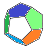MB Matheblog # 17 blog overwiewprevious article main pageindex website

 2022-02-07deutsche Version

Introduction

In figure 1 we see 2D regular grids. These are regular rectangular tesselations built from $$~n~$$ congruent rectangles. The left grid in figure 1 is special because the tesselation is built from squares; these grids are called 2D Cartesian grids or aa-grids. The right grid in figure 1 is non-cartesian and called an ab-grid, being built from rectangles with different edge lengths and equal orientation.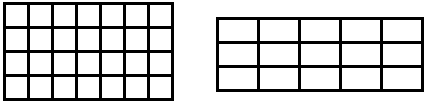Figure 1  2D regular grids; the left one is an aa-grid (Cartesian), the right one is an ab-grid

We should mention another (equivalent) definition of the tesselations in figure 1. The Cartesian grid can be seen as a rectangle with integer edge lengths and area $$~n~$$.  The non-Cartesian grid can be seen as a rectangle with edge lengths $$~(m_1,~m_2 \cdot b)~$$ and irrational $$~b~$$,  or with edge lengths $$~(m_1 \cdot a,~m_2 \cdot b)~$$ and incommensurable $$~a,~b~$$.  In both cases is $$~m_1 \cdot m_2=n~$$ with integer $$~m_1,~m_2~$$.  –  With this definition the tesselation borders inside the outer rectangle can be omitted.

In figure 2 we see 3D regular grids. These are regular honeycombs of boxes built from $$~n~$$ congruent boxes with equal orientation. The left grid in figure 2 is built from cubes and called 3D Cartesian grid or aaa-grid. The grid in the middle is called aab-grid, the building blocks having edges with two different lengths. The right grid is called abc-grid, the building blocks having edges with three different lengths.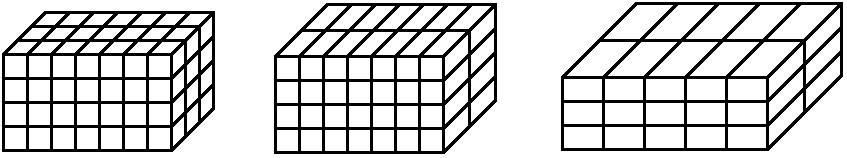Figure 2  3D regular grids; from left to right: aaa-grid (Cartesian), aab-grid, abc-grid

An equivalent definition of the honeycombs in figure 2, similar to the 2D case, can be given. The Cartesian grid can be seen as a box with integer edge lengths and volume $$~n~$$.  The aab-grid can be seen as a box with edge lengths $$~(m_1,~m_2,~m_3 \cdot b)~$$ and irrational $$~b~$$,  or with edge lengths $$~(m_1 \cdot a,~m_2 \cdot a,~m_3 \cdot b)~$$ and incommensurable $$~a,~b~$$.  The abc-grid can be seen as a box with edge lengths $$~(m_1,~m_2 \cdot b,~m_3 \cdot c)~$$ and irrational incommensurable $$~b,~c~$$ or with edge lengths $$~(m_1 \cdot a,~m_2 \cdot b,~m_3 \cdot c)~$$ and incommensurable $$~a,~b,~c~$$.  In all these cases is $$~m_1 \cdot m_2 \cdot m_3=n~$$ with integer $$~m_1,~m_2,~m_3~$$.  –  With this definition the honeycomb borders inside the outer box can be omitted.

The following sections deal with the number of ways to arrange $$~n~$$ identical rectangles or boxes in regular grids, modulo rotation.

Divisors

We want to give a formula for the number of divisors of a natural number $$~n~$$.  This will be an essential tool for solving the problems presented at the end of the introduction. We write $$~n~$$ as the unique product of its prime factors $$~p_i~$$: $\textbf{(1)}~~~n=\prod_i p_i^{h_i}$ The divisors of $$~n~$$ can contain each prime factor $$~p_i~$$ with power $$~0~...~h_i~$$.  So there are $$~1+h_i~$$ ways for $$~p_i~$$ to appear (to some power, including $$~0$$) in a divisor of $$~n~$$.  The number of divisors of $$~n~$$ (including $$~1~$$ and $$~n~$$) will be denoted by $$~\tau(n)~$$ : $\textbf{(2)}~~~\tau(n)=\prod_i ~(1+h_i)$ Remark: $$~n=\prod_i~ p_i^{h_i}~$$ can also be read as an infinite product containing all primes $$~p_i~$$ if we allow for $$~h_i=0~$$.  This gives a bijection between the set $$~\textbf{N}~$$ of natural numbers and the sequence space $$~\textbf{N}_{\text{o}}^{\infty}~$$ of all "finite" sequences of non-negative integers by the mapping $$~n \longleftrightarrow (h_i)_{i \in \textbf{N}}~$$,  "finite" meaning "containing finitely many non-zero members". Thus $$~\textbf{N}_{\text{o}}^{\infty}~$$ is countable.

Mathematica:
$$~p_i~$$ and $$~h_i~$$ are given by  FactorInteger[n]
$$~\tau(n)~$$ is given by  Length[Divisors[n]]
or  f=FactorInteger[n]; le=Length[f]; Product[1+f[[m]][],{m,le}]

OEIS:
$$\tau(n)~$$ is given by  A000005.

2D grids (aa- and ab-grids)

The Cartesian grid in the next figure contains $$~n=28~$$ squares and is a $$~(4 \times 7)-$$grid.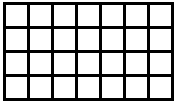We see that $$~4 \times 7~$$ can be replaced by $$~m_1 \times m_2~$$ with $$~m_1,~m_2~$$ divisors of $$~n~$$.  We want to count all ways for building these grids: As $$~m_2 = n/m_1~$$ and $$~(m_1 \times m_2)-$$grids are identified with $$~(m_2 \times m_1)-$$grids, $$~m_1~$$ can run through half of the divisors  –  but only if $$~n~$$ is not a square. If $$~n~$$ is a square number then a $$~(\sqrt{n} \times \sqrt{n})~-$$grid exists; this is the only case for the number of divisors being odd. We denote the number of 2D Cartesian grids with $$~n~$$ squares by $$~g_{aa}(n)~$$ and get: $\textbf{(3)}~~~g_{aa}(n)=\left\lceil \frac{\tau (n)}{2} \right\rceil$ Remarks:
For $$~\tau(n)~$$ see (2).
$$\lceil~..\rceil$$ gives the smallest integer greater than or equal to the argument $$~~~\longrightarrow~~~g_{aa}(n)=(\tau(n)+1)/2~$$ if $$~n~$$ is a square and $$~g_{aa}(n)=\tau(n)/2~$$ otherwise.

Mathematica:
$$g_{aa}(n)~$$ is given by  Ceiling[Length[Divisors[n]]/2]

OEIS:
$$g_{aa}(n)~$$ is given by  A038548.

The non-Cartesian grid in the next figure contains $$~n=15~$$ rectangles and is a $$~(3 \times 5)-$$grid.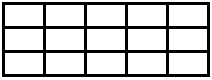$$3 \times 5~$$ can be replaced by $$~m_1 \times m_2~$$ with $$~m_1,~m_2~$$ divisors of $$~n~$$.  But in contrast to Cartesian grids $$~(m_1 \times m_2)-$$grids cannot be identified with $$~(m_2 \times m_1)-$$grids. So $$~m_1~$$ can run through all divisors. We denote the number of 2D non-Cartesian grids with $$~n~$$ rectangles by $$~g_{ab}(n)~$$ and get:

$$\textbf{(4)}~~~g_{ab}(n)=\tau (n)~$$,  cf. (2)

3D Cartesian grids (aaa-grids)

The Cartesian grid in figure 3 contains $$~n=84~$$ cubes and is a $$~(4 \times 7 \times 3)-$$grid.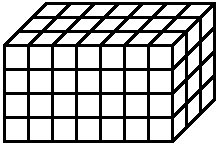Figure 3  aaa-grid

As in the similar 2D case $$~(m_1 \times m_2 \times m_3)-$$grids are identified with $$~(m_1 \times m_3 \times m_2)-$$grids, and so on for other permutations of the three divisors. So we look for divisors satisfying $$~m_1 \leq m_2 \leq m_3~$$. We denote the number of 3D Cartesian grids made from $$~n~$$ cubes by $$~g_{aaa}(n)~$$.  We know no formula for $$~g_{aaa}(n)~$$.

Mathematica:
$$g_{aaa}(n)~$$ is given by
b=0; d=Divisors[n]; r=Length[d];
Do[If[d[[h]] d[[i]] d[[j]]==n, b++], {h, r}, {i, h, r}, {j, i, r}];
b

OEIS:
$$g_{aaa}(n)~$$ is given by  A034836.

aab-grids

The non-Cartesian $$~(4 \times 7 \times 2)-$$grid in figure 4 is an aab-grid containing $$~n=56~$$ congruent boxes with edges of two different lengths.Figure 4  aab-grid

An easy way for determining the number of different aab-grids is to add up all Cartesian 2D grids  –  the "front" of the box in figure 4 (with squares) may serve as an example. What does "all" mean here? The 2D grid must contain $$~m~$$ squares with $$~m|n~$$ ($$m~$$ is a divisor of $$~n$$). We denote the number of aab-grids with $$~n~$$ boxes by $$~g_{aab}(n)~$$.  Using (3) we get: $\textbf{(5)}~~~g_{aab}(n)=\sum_{m|n} g_{aa}(m)=\sum_{m|n}~\left\lceil \frac{\tau (m)}{2} \right\rceil$ Mathematica:
$$g_{aab}(n)~$$ is given by
d=Divisors[n]; r=Length[d];
Sum[Ceiling[Length[Divisors[d[[j]]]]/2],{j, r}]

OEIS:
$$g_{aab}(n)~$$ is given by  A140773.

abc-grids

The non-Cartesian $$~(3 \times 5 \times 2)-$$grid in figure 5 is an abc-grid containing $$~n=30~$$ congruent boxes with edges of three different lengths.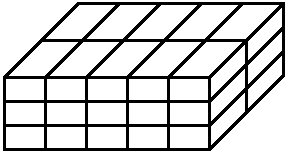Figure 5  abc-grid

We denote the number of abc-grids with $$~n~$$ boxes by $$~g_{abc}(n)~$$.

We want to show that $$~g_{abc}(n)~$$ equals the number of divisors of divisors of $$~n~$$. This means we have to check each divisor $$~m~$$ of $$~n~$$ and determine the number $$~\tau (m)~$$ of divisors of $$~m~$$.  All these numbers add up to $$~g_{abc}(n)~$$.

Look at one of the faces of the box in figure 5, e.g. the "front" with $$~15~$$ rectangles. The number of these rectangles must be a divisor of $$~n~$$,  and each divisor of $$~n~$$ must be considered because the faces are non-Cartesian 2D grids; cf. the explanations leading to (4). Let $$~m~$$ be such a divisor. $$~m~$$ stands for the number of rectangles in a $$~(k \times m/k)-$$grid with $$~k|m~$$.  So for each divisor $$~m~$$ of $$~n~$$ we get $$~\tau (m)~$$ different abc-grids. This settles our claim.

What was shown amounts to the realization that we need to look at only one face of the box  —  as the number $$~n/m~$$ of layers "behind" it is determined by the number $$~m~$$ of rectangles on the face. This number is a divisor $$~m~$$ of $$~n~$$,  and $$~m~$$ can be split into $$~k \cdot (m/k)~$$ with $$~k|m~$$ in order to build a rectangle for the face. We get a $$~(m_1 \times m_2 \times m_3)-$$grid with $$~(m_1 \times m_2 \times m_3)=(k \times (m/k) \times (n/m))~$$ where any permutation of the $$~m_i~$$ results in a different grid.

$$m|n~$$ can be written as $$~m=\prod_{i=1}^s p_i^{v_i}~$$ with $$~0 \leq v_i \leq h_i~$$,  see (1), and any such tuple $$~(v_i)_{~i=1~..~s}~$$ gives a divisor $$~m~$$ of $$~n~$$.  $$~m~$$ has $$~\tau(m)=\prod_{i=1}^s~(1+v_i)~$$ divisors, see (2). With this we get: $g_{abc}(n)=\sum_{0\leq v_i \leq h_i}~\prod_{i=1}^s ~(1+v_i)=\sum_{v_1=0}^{h_1}~\sum_{v_2=0}^{h_2}~...\sum_{v_s=0}^{h_s}~\prod_{i=1}^s ~(1+v_i)=\sum_{v_1=0}^{h_1}(1+v_1) \sum_{v_2=0}^{h_2}(1+v_2)~...\sum_{v_s=0}^{h_s}(1+v_s)$ $\textbf{(6)}~~~g_{abc}(n)=\prod_{i=1}^s~\binom{2+h_i}{2}$ Mathematica:
$$g_{abc}(n)~$$ is given by  Length[Flatten[Divisors[Divisors[n]]]]
or  f=FactorInteger[n]; r=Length[f]; Product[Binomial[2+f[[m]][],2],{m, r}]

OEIS:
$$g_{abc}(n)~$$ is given by  A007425.